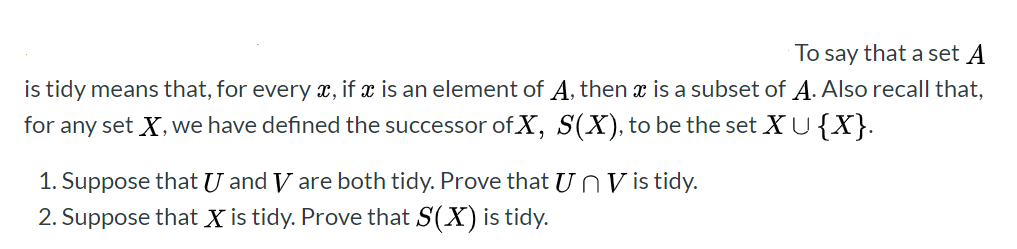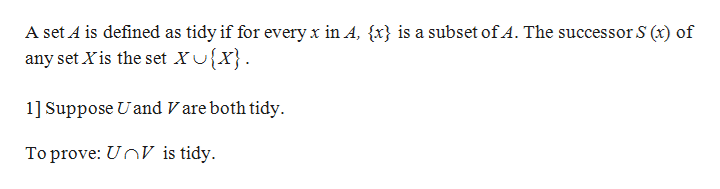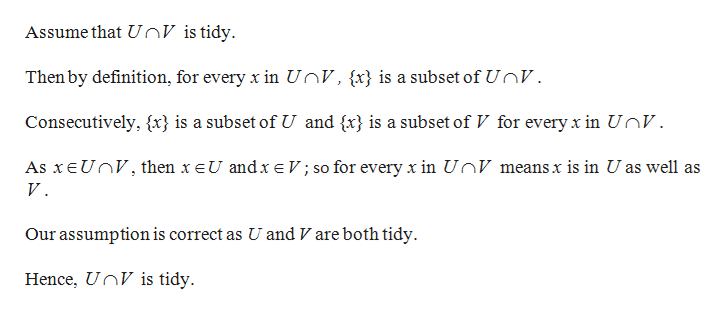# To say that a set Ais tidy means that, for every x, if x is an element of A, thenis a subset of A. Also recall that,for any set X, we have defined the successor ofX, S(X), to be the set XU{X}.1. Suppose that U and V are both tidy. Prove that U n V is tidy.2. Suppose that X is tidy. Prove that S(X) is tidy.

Question
110 views

To say that a set A is tidy means that, for every x, if x is an element of A, then x is a subset of A. Also recall that, for any set X, we have defined the successor of X, S ( X ), to be the set X ∪ { X }.

1. Suppose that U and V are both tidy. Prove that U ∩ V is tidy.
2. Suppose that X is tidy. Prove that S ( X ) is tidy.help_outlineImage TranscriptioncloseTo say that a set A is tidy means that, for every x, if x is an element of A, then is a subset of A. Also recall that, for any set X, we have defined the successor ofX, S(X), to be the set XU{X}. 1. Suppose that U and V are both tidy. Prove that U n V is tidy. 2. Suppose that X is tidy. Prove that S(X) is tidy. fullscreen
check_circle

Step 1

Given:help_outlineImage TranscriptioncloseA set A is defined as tidy if for every x in A, {x} is a subset of A. The successor S (x) of any set Xis the set X0{x}. 1] Suppose Uand V are both tidy To prove: UnV is tidy. fullscreen
Step 2

Proof:help_outlineImage TranscriptioncloseAssume that UnV is tidy Then by definition, for every x in UnV, {x} is a subset of UnV Consecutively, {x} is a subset of U and {x} is a subset of V for every x in UnV. As xE UnV, then x eU andx e V; so for everyx in UnV means x is in U as well as V Our assumption is correct as U and V are both tidy Hence, UnV is tidy. fullscreen
Step 3

2] Suppose X is t...

### Want to see the full answer?

See Solution

#### Want to see this answer and more?

Solutions are written by subject experts who are available 24/7. Questions are typically answered within 1 hour.*

See Solution
*Response times may vary by subject and question.
Tagged in

### Math